# 层次分析法（AHP）——matlab代码实现

层次分析法（AHP）的主要思想是根据研究对象的性质将要求达到的目标分解为多个组成因素，并按组成因素间的相互关系，将其层次化，组成一个层次结构模型，然后按层分析，最终获得最高层的重要性权值。层次分析法把一个复杂的无结构问题分解组合成若干部分或若干因素，上一层次对相邻的下一层次的全部或某些元素起支配作用，这样就形成了自上而下的层次结构，通过相关指标之间的两两比较对系统中各指标进行优劣判断，利用判断结果来综合计算各指标间的权重，从而对主要的影响因素进行排序。基本流程如下：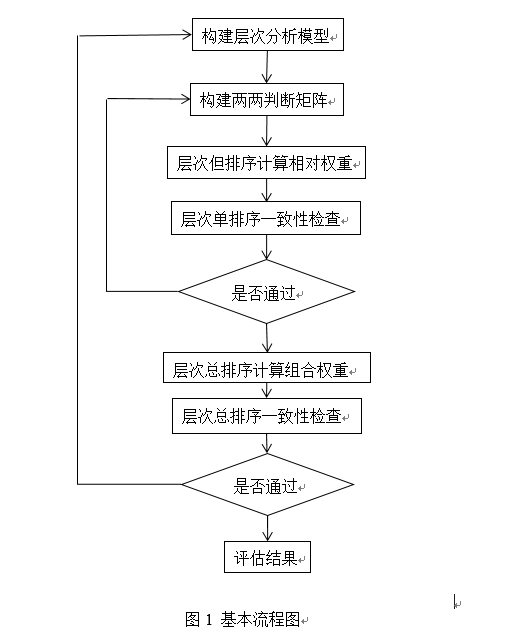1.建立层次结构模型

2.建立判断矩阵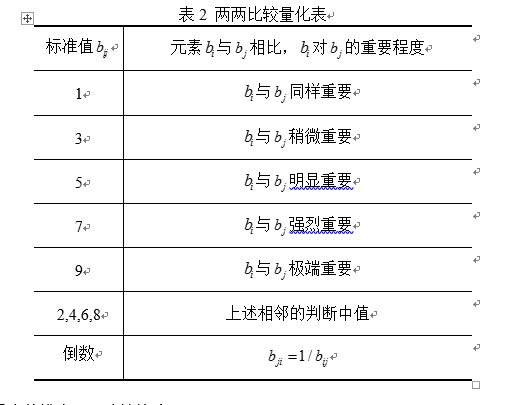3.层次单排序和一致性检验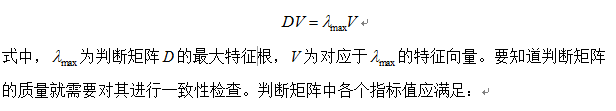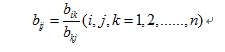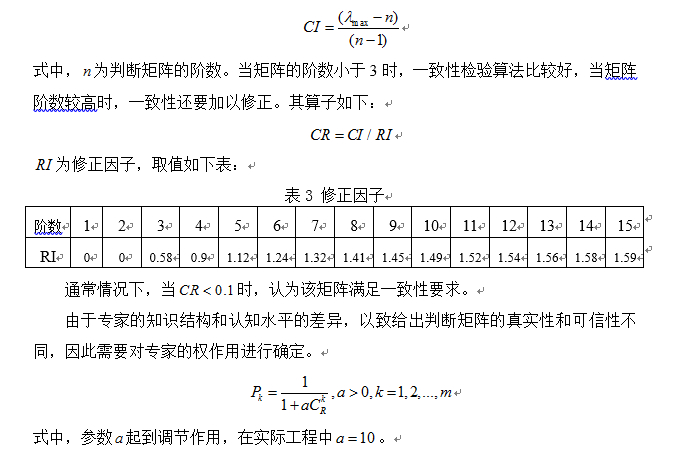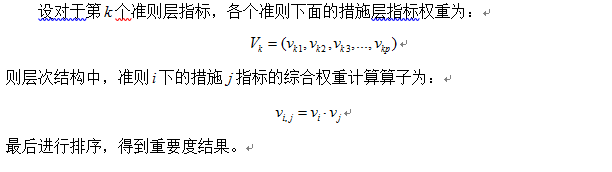function [Q]=AHP(B)
%Q为权值，B为对比矩阵
%导入判别矩阵B
[n,m]=size(B);
%判别矩阵具有完全一致性
for i=1:n
for j=1:n
if B(i,j)*B(j,i)~=1
fprintf('i=%d,j=%d,B(i,j)=%d,B(j,i)=%d\n',i,j,B(i,j),B(j,i))
end
end
end
%求特征值特征向量,找到最大特征值对应的特征向量
[V,D]=eig(B);
tz=max(D);
tzz=max(tz);
c1=find(D(1,:)==max(tz));
tzx=V(:,c1);%特征向量
%权
quan=zeros(n,1);
for i=1:n
quan(i,1)=tzx(i,1)/sum(tzx);
end
Q=quan;
%一致性检验
CI=(tzz-n)/(n-1);
RI=[0,0,0.58,0.9,1.12,1.24,1.32,1.41,1.45,1.49,1.52,1.54,1.56,1.58,1.59];
%判断是否通过一致性检验
CR=CI/RI(1,n);
if CR>=0.1
fprintf('没有通过一致性检验\n');
else
fprintf('通过一致性检验\n');
end

end

10-171万+08-165万+
07-271048
11-226752
07-062717
04-122579
09-18
04-193239
07-03
12-202万+
©️2020 CSDN 皮肤主题: 大白 设计师:CSDN官方博客